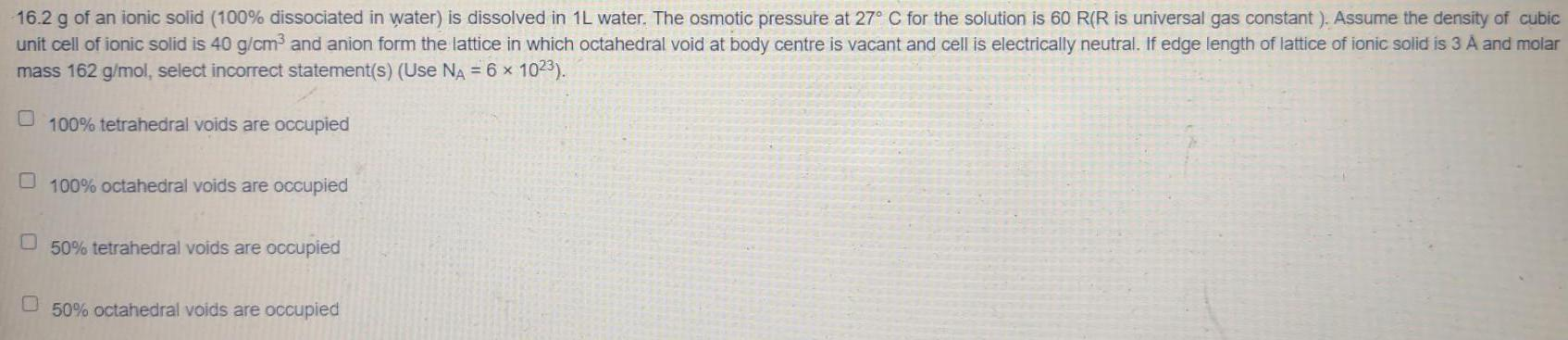Question:

# 16.2 g of an ionic solid (100% dissociated in water) is

Last updated: 7/8/202216.2 g of an ionic solid (100% dissociated in water) is dissolved in 1L water. The osmotic pressure at 27° C for the solution is 60 R(R is universal gas constant). Assume the density of cubic unit cell of ionic solid is 40 g/cm³ and anion form the lattice in which octahedral void at body centre is vacant and cell is electrically neutral. If edge length of lattice of ionic solid is 3 A and molar mass 162 g/mol, select incorrect statement(s) (Use NA = 6 × 1023). 100% tetrahedral voids are occupied 100% octahedral voids are occupied 50% tetrahedral voids are occupied 50% octahedral voids are occupied